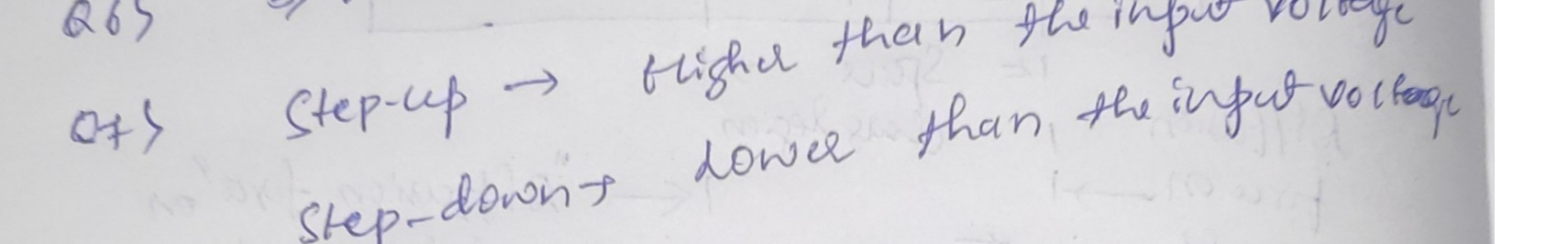Question

Robotics

A Transformer, is a device that transfers electric energy from one alternating-current circuit to one or more other circuits

The output voltage in a step-down transformer is

The output voltage in a step-up transformer isVerified### Question 45481Robotics

Given in the figure below the response of a thermocouple in time domain. The x-axis represents time in (ms) and the y-axis represents voltage in (mV).
a) Find the time constant.
b) Find the response time.
c) What is the relation between the time constant and the response time? (1 mark)

### Question 45479Robotics

A water main of a circular cross-sectional area 5.50 m² has water flowing through it. It was noticed that the water was not flowing at normal speed and the testing engineer decided to use Hall sensor to measure the velocity of water. A magnetic field is applied across the main of value 0.756 uT. The sensor reads a Hall emf of value 2.75 µV.
a) What is the velocity of water if the flow is perpendicular to the applied field. (1.5 marks)
b) What is the percentage reduction in the velocity of the flow if under normal conditions,water moves from point A to B in 0.5 s.(2.5 marks)

### Question 41850Robotics

Figure (a) below shows the construction of a tunable capacitor that is actuated by using electrostatic force between the high-aspect ratio, inter digitated fingers. All the fingers connected to port-1 are free to move in the direction shown in Figure (a), and all the fingers that are connected to port-2 are stationery. Port-2 fingers are suspended bya set of springs, and an overall spring constant of k is defined for lumped modeling. Figure (b) shows the details ofa stationery-movable finger arrangement with the design geometries. Note that the overall tunable capacitor is the arca modulated capacitor (CI) and there is an overall parasitic capacitor at the cnd of the finger modeled with Cp.Number of finger pairs is N, the device is operating in air, heavily damped and all the fringing capacitances can be ignored. Find symbolic expressions in (a), (b), (c) as a function of geometries and k.
(a) Find expressions for Cp and C1. Assuming stationery values for these caps as CPo and C10, find the tendency of these caps with changes in displacement in r-direction.
(b) Assume a voltage drive between ports I and 2. What is the maximum stable displacement xmax of the device?
(c) Find the maximum value of the tuning capacitance for stable actuation.
(d) Use N = 300, one-side length of one of the springs' length (i.e. from the anchor to the truss) L = 500 um,spring width w = 1 µm, d= 2 µm, h = 50 um, wf = 4 µm, xf = 30 um, re = 10 µm. Calculate the values of Cpo and C10, the maximum stable displacement and the maximum value of the tuning capacitance Cimaz-

### Question 38800Robotics

If you have a microcontroller and actuator, you need to have one of the following so the microcontroller can communicate properly with the actuator.
O a. Graphical interface
O b. Input signal conditioning and interfacing
O c. Output signal conditioning and interfacing
O d. Digital architecture

### Question 38799Robotics

Computer CPU has three major components, (A) Arithmetic Logic Unit, (B) Memory Unit and (C)Input Unit?
D True
O False

### Question 38798Robotics

When converting the binary number 11111111 into decimal the answer is ?
C. 230
a. 156
O b. 255
O d. 303

### Question 38797Robotics

A Transformer, is a device that transfers electric energy from one alternating-current circuit to one or more other circuits
The output voltage in an isolation transformer is
The output voltage in an isolation transformer is

### Question 38796Robotics

The conversion of 16 decimal to binary is?
a, 100
C. 1000
O d. 10000
b. 00001

### Question 38795Robotics

In the following logic gates, the value of input B is?
O b. Either 0 or 1
O c.0 only
O d. 1 only

### Question 38794Robotics

The binary number equivalent to 413 decimal is?
O c. None of the answers
O b. 110011 101
a. 10011110100
O d. 100111100

### Submit query